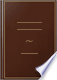Søk Bilder Maps Play YouTube Nyheter Gmail Disk Mer »
Logg på
 Bøker Bok 1–10 av 107 på In a right triangle, the side opposite the right angle is called the hypotenuse and...In a right triangle, the side opposite the right angle is called the hypotenuse and is the longest side.The measure of the circle, perfected in January, 1845 - Side 71
av John Davis (of Providence, R.I.) - 1854
Uten tilgangsbegrensning - Om denne boken## The New Practical Navigator: Being a Complete Epitome of Navigation: to ...

John Hamilton Moore - 1810 - 336 sider
...acute-angled triangle. XV. A RIGHT-ANGLED TRIANGLE bas one of its angles right, or containing 90 degrees ; the side opposite the right angle is called the hypotenuse, and the other two sides are called legs ; that which stands upright is called the perpendicular, and the other tne base : thus...
Uten tilgangsbegrensning - Om denne boken## A treatise on navigation, and nautical astronomy

Edward Riddle - 1824
...arc. V "" \ 51. A Quadrant is a sector whose bounding radii are perpendicular to each other. 52. In a right angled triangle, the side opposite the right angle is called the Hypolhenuse, and the side including the right angle are called the Base and Perpendicular, and sometimes...
Uten tilgangsbegrensning - Om denne boken## The New American Practical Navigator: Being an Epitome of Navigation ...

Nathaniel Bowditch - 1826 - 617 sider
...obtuse-angled triangle, and an acute angled triangle. XV. A RIGHT-ANGLED TRIANGLE has one of its angles right : the side opposite the right angle is called the hypotenuse ; and the other two sides are called legs ; that which stands upright, is called the perpendicular, and the other the ¿eat ;...
Uten tilgangsbegrensning - Om denne boken## Elements of Geometry: With Notes

John Radford Young - 1827 - 208 sider
...which has all its sides equal. 17. A right angled triangle is one which has a right angle. 18. In a right angled triangle the side opposite the right angle is called the hypothenuse. If, for example, the angle A is right, the side BC is the hypothenuse. Any side of a triangle...
Uten tilgangsbegrensning - Om denne boken## The Young Man's Book of Knowledge: Containing a Familiar View of the ...

Thomas Tegg - 1827 - 348 sider
...which has only two equal sides, as BEF. HI. A right-angled Triangle has one of its E angles right ; the side opposite the right angle is called the hypotenuse, and the oilier two sides arc called legs ; that which Mauds upright is called the perpendicular, and the other...
Uten tilgangsbegrensning - Om denne boken## The Elements of Euclid: viz. the first six books, together with the eleventh ...

Euclid, Robert Simson - 1835 - 513 sider
...cosine, tangent, radius, &c. respectively. NOTE 2. In a right angled triangle, the side subtending the right angle is called the hypotenuse ; and the other two sides which contain the right angle are called the legs ; one of the legs is also called the perpendicular,...
Uten tilgangsbegrensning - Om denne boken## The Elements of Euclid: viz. the first six books, together with the eleventh ...

Euclid, Robert Simson - 1835 - 513 sider
...cosine, tangent, radius, &c. respectively. NOTE 2. In a right angled triangle, the side subtending the right angle is called the hypotenuse ; and the other two sides which contain the right angle are called the legs ; one of the legs is also called the perpendicular,...
Uten tilgangsbegrensning - Om denne boken## The Mechanic's Calculator: Comprehending Principles, Rules, and Tables in ...

William Grier - 1839 - 308 sider
...let fall from an angle, or its vertex, to the opposite side, called the base. 55. In a right-angled triangle, the side opposite the right angle is called the Hypotenuse ; and the other two sides are called the Legs, and sometimes the Base and Perpendicular. 56. When an angle is denoted by three...
Uten tilgangsbegrensning - Om denne boken## The Mechanic's Calculator: Comprehending Principles, Rules, and Tables in ...

William Grier - 1842 - 308 sider
...>«. or its vertex, to the opposite side, called j/ IN. the base. ^- '—^ • 55. In a right-angled triangle, the side opposite the right angle is called the Hypotenuse ; and the other two sides are called the Legs, and sometimes the Base and Perpendicular. jj v 56. When an angle is denoted by...
Uten tilgangsbegrensning - Om denne boken## Elements of plane (solid) geometry (Higher geometry) and trigonometry (and ...

1845
...triangle which has one obtuse angle. 26. A right angled triangle is onf. which has a right angle. 27. In a right angled triangle, the side opposite the right angle is called the /lypothemtse. If, for example, the angle A is right, the side B(J is the hypothennse. Any side of a...
Uten tilgangsbegrensning - Om denne boken